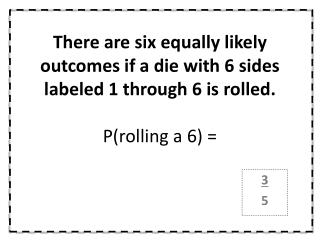DownloadDownload Presentation3 5

# 3 5

Download Presentation## 3 5

- - - - - - - - - - - - - - - - - - - - - - - - - - - E N D - - - - - - - - - - - - - - - - - - - - - - - - - - -
##### Presentation Transcript

1. There are six equally likely outcomes if a die with 6 sides labeled 1 through 6 is rolled.P(rolling a 6) = 3 5

2. There are six equally likely outcomes if a die with 6 sides labeled 1 through 6 is rolled.What is the complement of rolling a 6? 1 4

3. There are six equally likely outcomes if a die with 6 sides labeled 1 through 6 is rolled.P(rolling a multiple of 2) = 1 3

4. A bag contains 5 blue, 8 red, and 7 green marbles. A marble is selected at random. Find the probability of each event.P(not blue) = 1 5

5. A bag contains 5 blue, 8 red, and 7 green marbles. A marble is selected at random. Find the probability of each event.P(not red or green) = 1 6

6. A bag contains 5 blue, 8 red, and 7 green marbles. A marble is selected at random. Find the probability of each event.P(green) = 3 4

7. Find the probability of randomly choose the letter p in the word “apple.” 2 3

8. You’re playing Rock Paper Scissors with your friend. What is the probability that your friend will throw a paper? 3 10

9. You’re playing Rock Paper Scissors with your friend. What is the probability that your friend will NOT throw a rock? 1 2

10. The spinner is spun one time. Find the probability of the eventP(divisible by 5) 5 6

11. The spinner is spun one time. Find the probability of the eventP(less than 15) 7 20

12. The spinner is spun one time. Find the probability of the eventP(a multiple of 3) 1